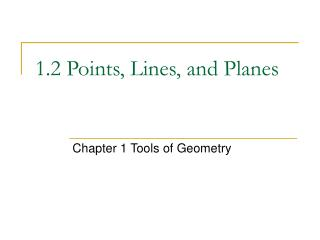DownloadDownload Presentation1.2 Points, Lines, and Planes

# 1.2 Points, Lines, and Planes

Télécharger la présentation## 1.2 Points, Lines, and Planes

- - - - - - - - - - - - - - - - - - - - - - - - - - - E N D - - - - - - - - - - - - - - - - - - - - - - - - - - -
##### Presentation Transcript

1. 1.2 Points, Lines, and Planes Chapter 1 Tools of Geometry

2. 1.2 Points, Lines, and Planes Point: a location; has no size; represented by a small dot and is named by a capital letter Space: set of all points Line: series of points that extends to two opposite directions Collinear Points: points that lie on the same line

3. n C F E m P Are points E, F, and C collinear? If so, name the line on which they lie. Are points E, F, and D collinear? If so, name the line on which they lie. Are points F, P, and C collinear? If so, name the line on which they lie. Name line m in three other ways. D l

4. Plane: Flat surface that has no thickness; contains many points and lines; named by a single capital letter or at least three of its noncollinear points Coplanar: points and lines in the same plane

5. H G Each surface of the ice cube represents part of a plane. Name the plane represented by the front of the ice cube. E F D C A B List three different names for the top of the ice cube.

6. Postulate or axiom: accepted statement of fact Postulate 1-1: Through any two points there is exactly one line Postulate 1-2: If two lines intersect, then they intersect in exactly one point. B A

7. Postulate 1-3: If two planes intersect, then they intersect in exactly one line. Postulate 1-4: Through any three noncollinear points there is exactly one plane.

8. H G What is the intersection of plane HGFE and plane BCGF? E F D C A B Name two planes that intersect in BF *Look in book at example 4, page 13

9. Homework Pg 13 1-37, 60-65